# 4. Plotting brain images¶

In this section, we detail the general tools to visualize neuroimaging volumes with nilearn.

Nilearn comes with plotting function to display brain maps coming from Nifti-like images, in the `nilearn.plotting` module.

Code examples

Nilearn has a whole section of the example gallery on plotting.

A small tour of the plotting functions can be found in the example Plotting tools in nilearn.

Finally, note that, as always in the nilearn documentation, clicking on a figure will take you to the code that generates it.

## 4.1. Different plotting functions¶

Nilearn has a set of plotting functions to plot brain volumes that are fined tuned to specific applications. Amongst other things, they use different heuristics to find cutting coordinates.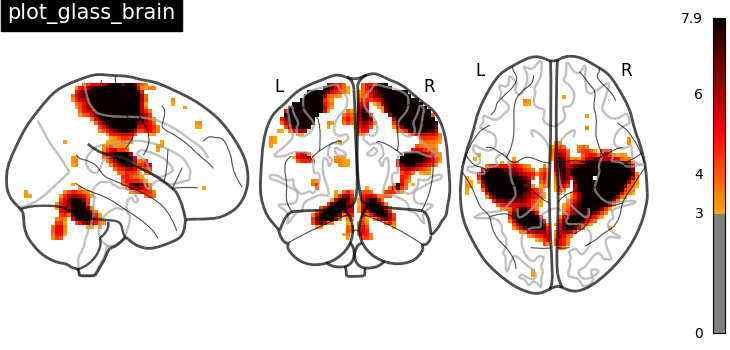`plot_anat`
Plotting an anatomical image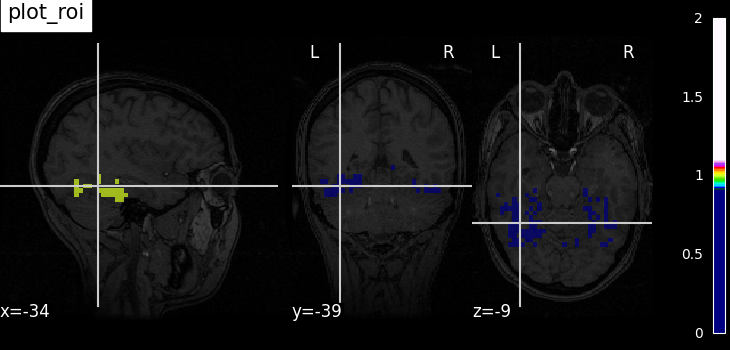`plot_epi`
Plotting an EPI, or T2* image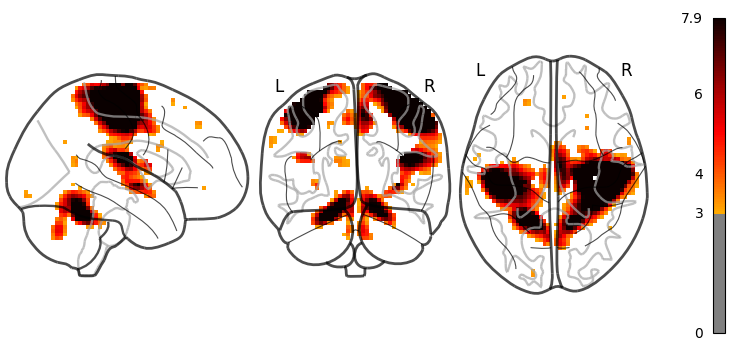`plot_glass_brain`
Glass brain visualization. By default plots maximum intensity projection of the absolute values. To plot positive and negative values set plot_abs parameter to False.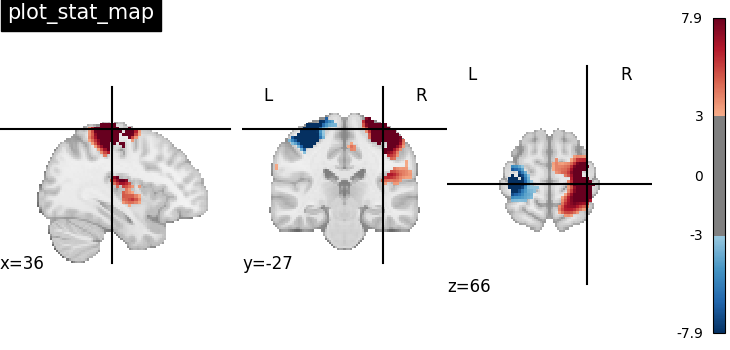`plot_stat_map`
Plotting a statistical map, like a T-map, a Z-map, or an ICA, with an optional background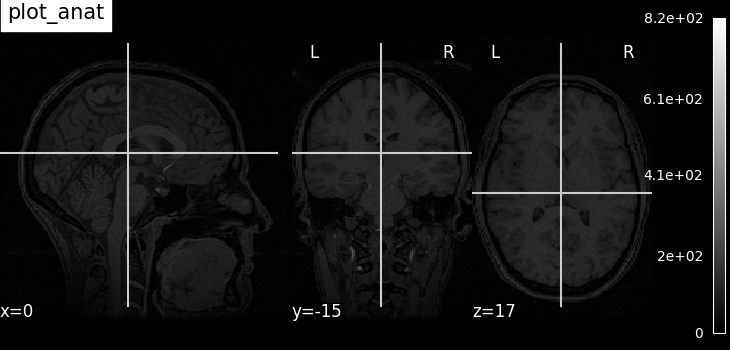`plot_roi`
Plotting ROIs, or a mask, with an optional background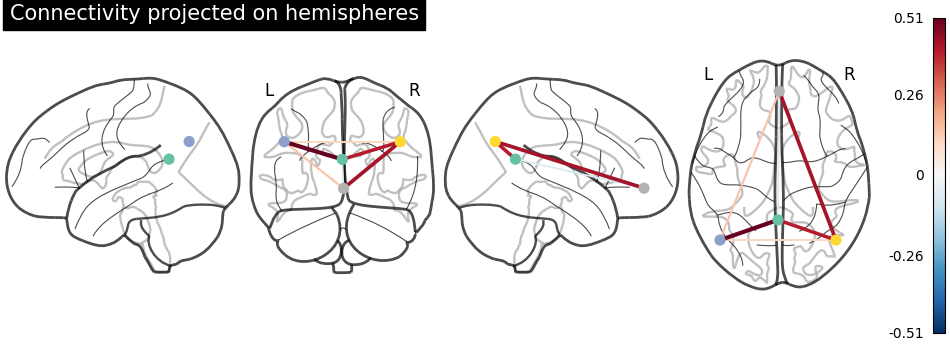`plot_connectome`
Plotting a connectome

Functions for automatic extraction of coords based on brain parcellations useful for `plot_connectome` are demonstrated in Example: Comparing connectomes on different reference atlases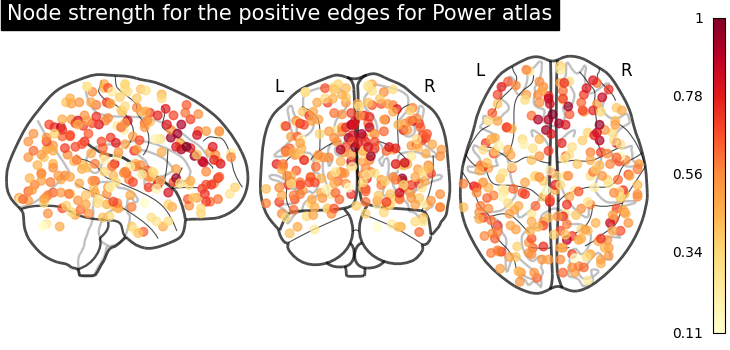`plot_markers`
Plotting network nodes (markers)

Function for automatic plotting of network nodes (markers) and color coding them according to provided nodal measure (i.e. connection strength) as demonstrated in Example: Comparing connectomes on different reference atlases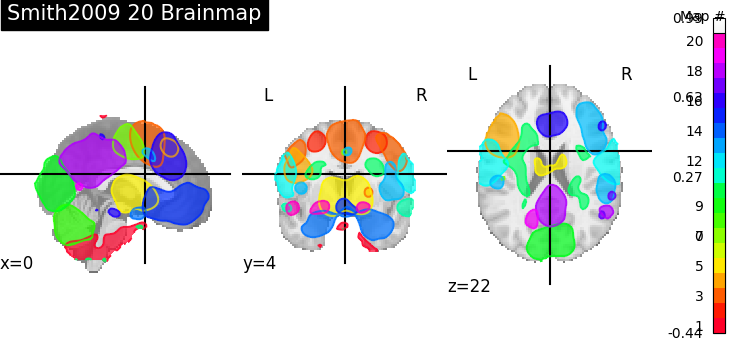`plot_prob_atlas`
Plotting 4D probabilistic atlas maps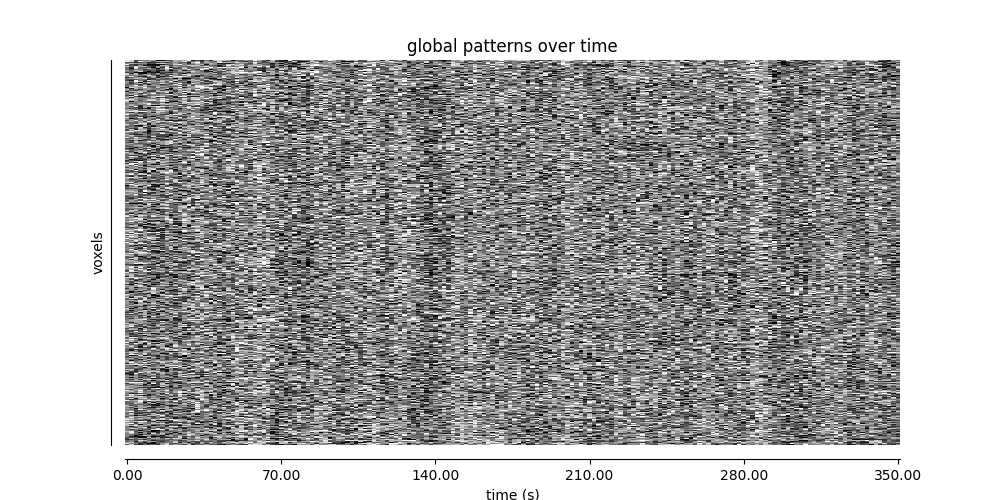`plot_carpet`
Plotting voxel intensities across time.

plot_img

`plot_img`
General-purpose function, with no specific presets

Warning

Opening too many figures without closing

Each call to a plotting function creates a new figure by default. When used in non-interactive settings, such as a script or a program, these are not displayed, but still accumulate and eventually lead to slowing the execution and running out of memory.

To avoid this, you must close the plot as follow:

```>>> from nilearn import plotting
>>> display = plotting.plot_stat_map(img)
>>> display.close()
```

## 4.2. Different display modes¶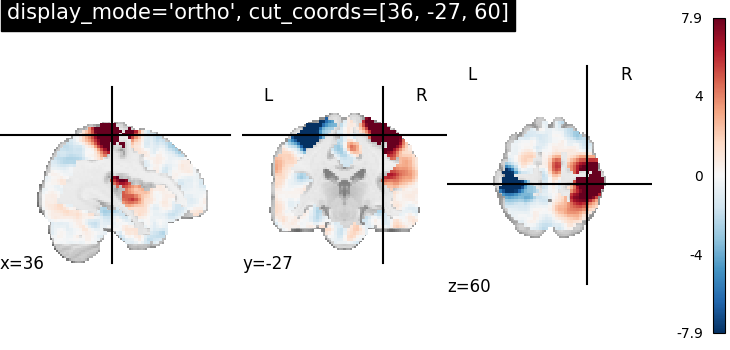display_mode=’ortho’, cut_coords=[36, -27, 60]
Ortho slicer: 3 cuts along the x, y, z directions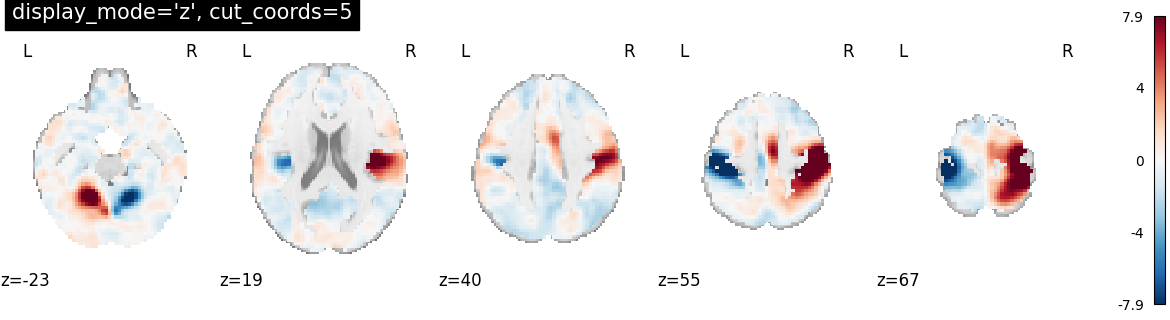display_mode=’z’, cut_coords=5
Cutting in the z direction, specifying the number of cuts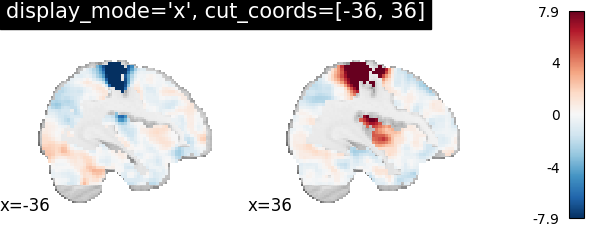display_mode=’x’, cut_coords=[-36, 36]
Cutting in the x direction, specifying the exact cuts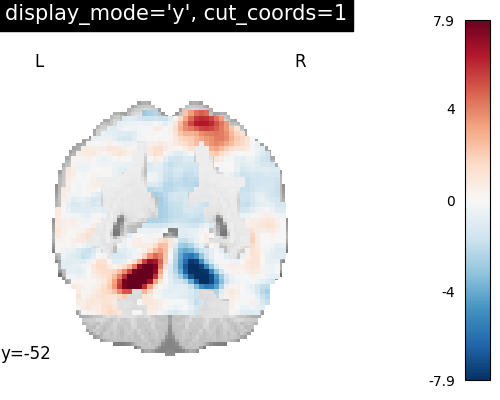display_mode=’y’, cut_coords=1
Cutting in the y direction, with only 1 cut, that is automatically positioneddisplay_mode=’z’, cut_coords=1, colorbar=False
Cutting in the z direction, with only 1 cut, that is automatically positioned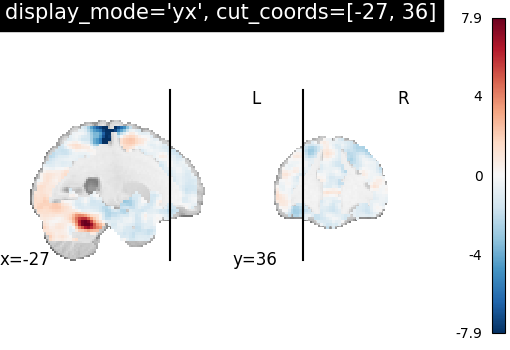display_mode=’xz’, cut_coords=[36, 60]
Cutting in the x and z direction, with cuts manually positioned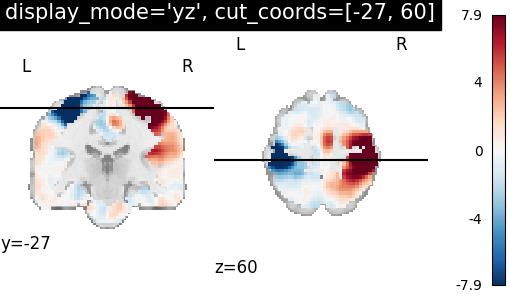display_mode=’yx’, cut_coords=[-27, 36]
Cutting in the y and x direction, with cuts manually positioned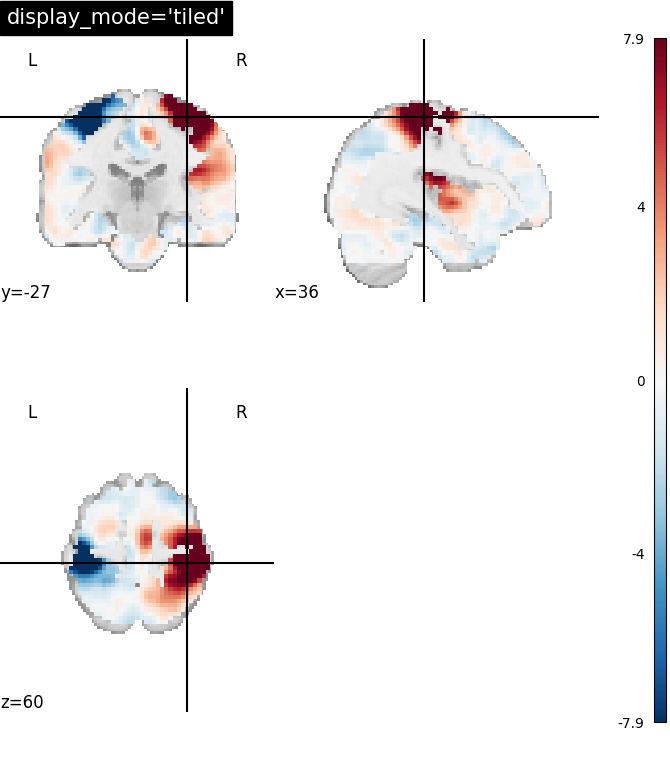display_mode=’yz’, cut_coords=[-27, 60]
Cutting in the y and z direction, with cuts manually positioned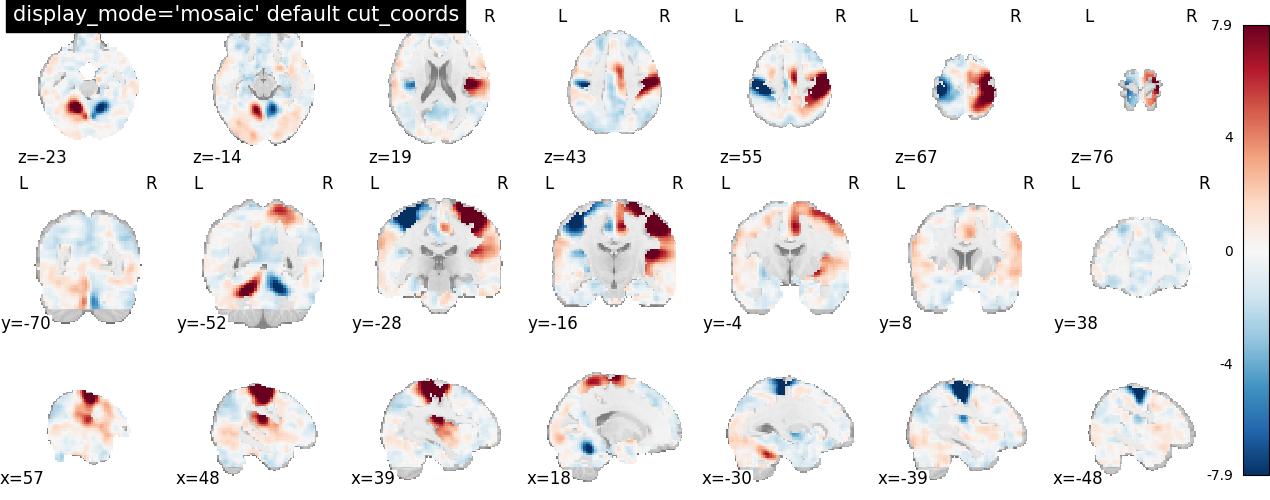display_mode=’tiled’, cut_coords=[36, -27, 60]
Tiled slicer: 3 cuts along the x, y, z directions, arranged in a 2x2 griddisplay_mode=’mosaic’
Mosaic slicer: multiple cuts along the x, y, z directions, with cuts automatically positioned by default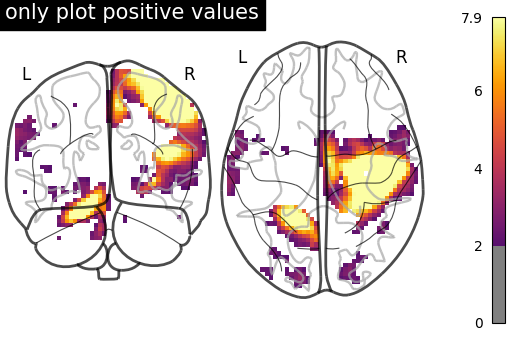Glass brain display_mode=’lzr’
Glass brain and Connectome provide additional display modes due to the possibility of doing hemispheric projections. Check out: ‘l’, ‘r’, ‘lr’, ‘lzr’, ‘lyr’, ‘lzry’, ‘lyrz’.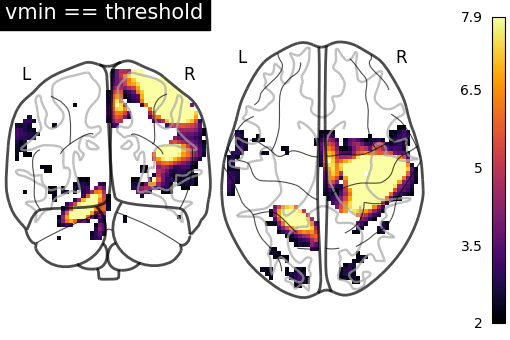Glass brain display_mode=’lyrz’
Glass brain and Connectome provide additional display modes due to the possibility of doing hemispheric projections. Check out: ‘l’, ‘r’, ‘lr’, ‘lzr’, ‘lyr’, ‘lzry’, ‘lyrz’.

## 4.3. Available Colormaps¶

Nilearn plotting library ships with a set of extra colormaps, as seen in the image below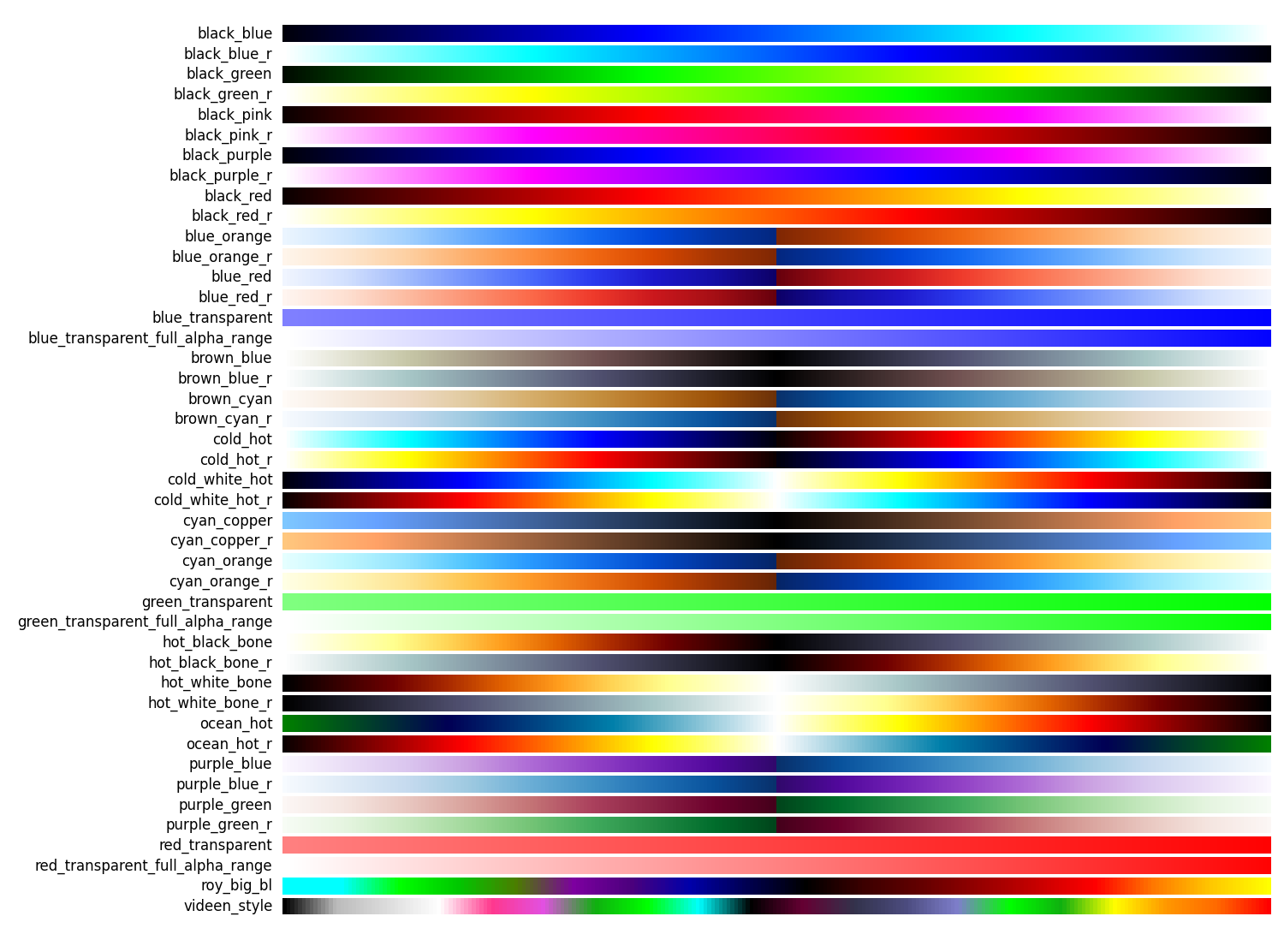These colormaps can be used as any other matplotlib colormap.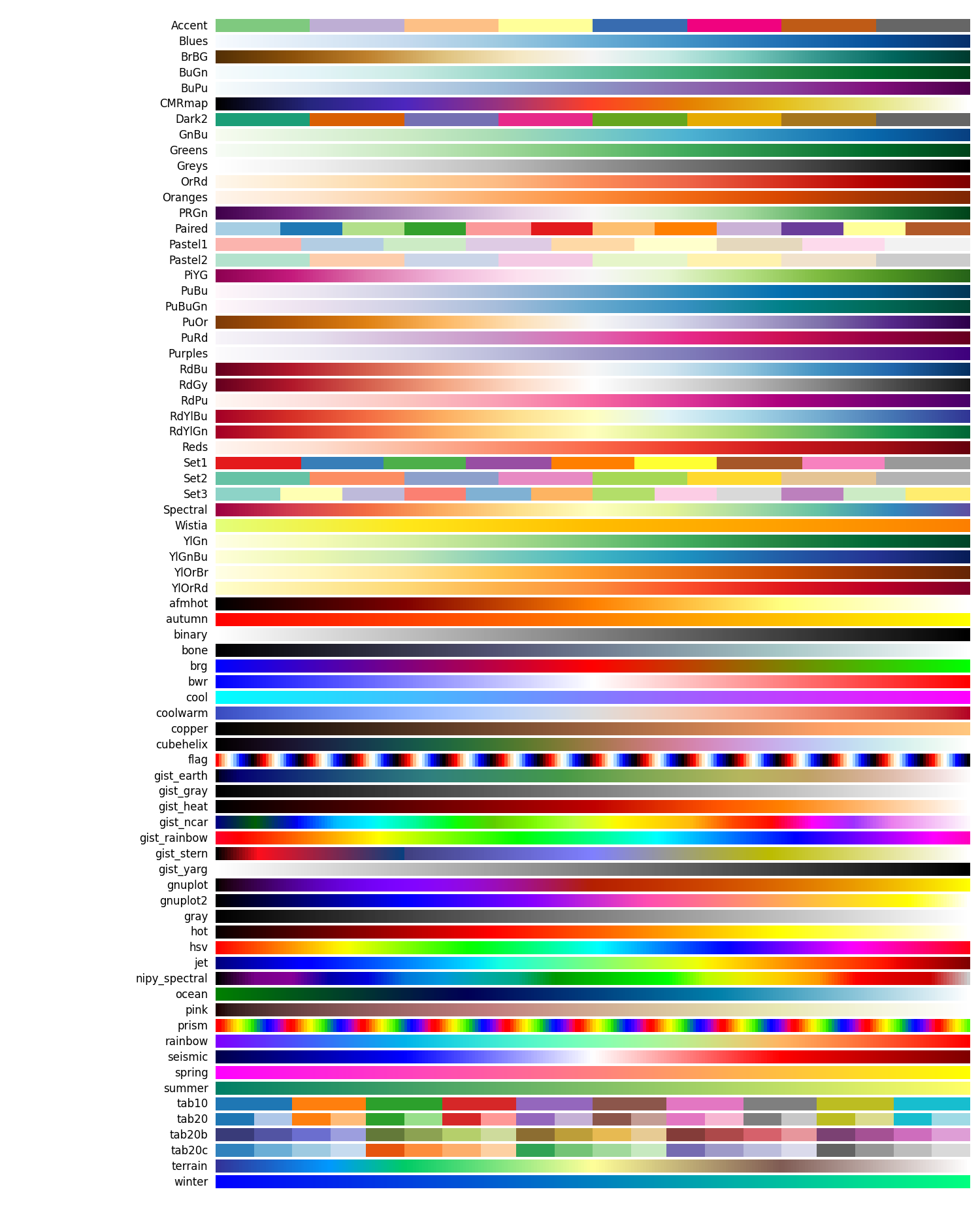## 4.4. Adding overlays, edges, contours, contour fillings, markers, scale bar¶

To add overlays, contours, or edges, use the return value of the plotting functions. Indeed, these return a display object, such as the `nilearn.plotting.displays.OrthoSlicer`. This object represents the plot, and has methods to add overlays, contours or edge maps:

```display = plotting.plot_epi(...)
```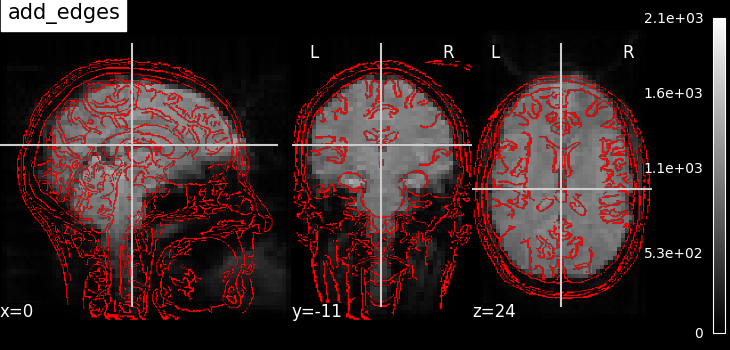Add a plot of the edges of img, where edges are extracted using a Canny edge-detection routine. This is typically useful to check registration. Note that img should have some visible sharp edges. Typically an EPI img does not, but a T1 does.Add a plot of the contours of img, where contours are computed for constant values, specified in ‘levels’. This is typically useful to outline a mask, or ROI on top of another map.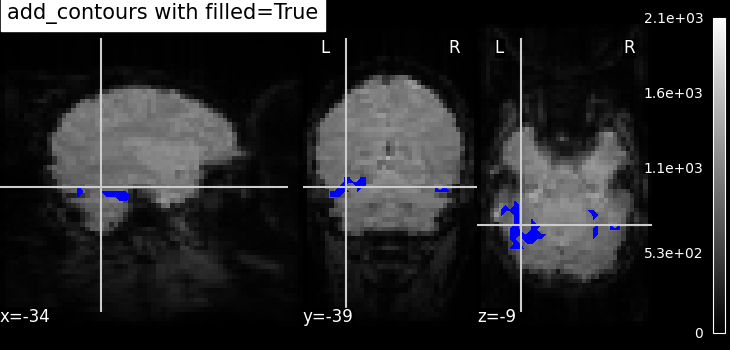Add a plot of img with contours filled with colors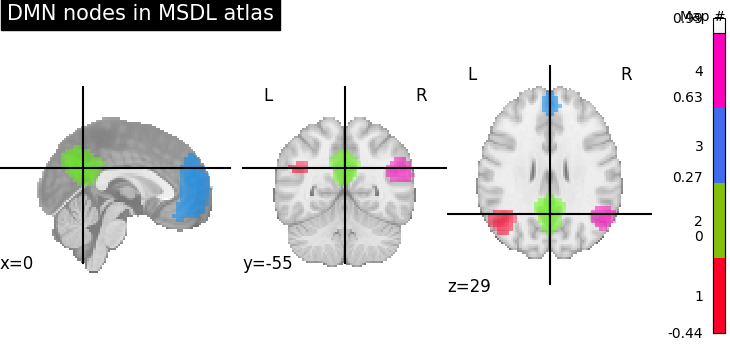Add a new overlay on the existing figure
Example: Visualizing a probabilistic atlas: the default mode in the MSDL atlasAdd seed based MNI coordinates as spheres on top of statistical image or EPI image. This is useful for seed based regions specific interpretation of brain images.
Example: Producing single subject maps of seed-to-voxel correlation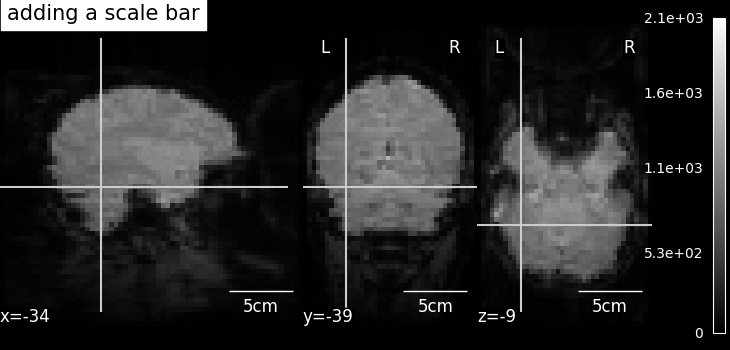display.annotate(scalebar=True)
Adds annotations such as a scale bar, or the cross of the cut coordinates
Example: More plotting tools from nilearn

## 4.5. Displaying or saving to an image file¶

To display the figure when running a script, you need to call `nilearn.plotting.show`: (this is just an alias to `matplotlib.pyplot.show`):

```>>> from nilearn import plotting
>>> plotting.show()
```

The simplest way to output an image file from the plotting functions is to specify the output_file argument:

```>>> from nilearn import plotting
>>> plotting.plot_stat_map(img, output_file='pretty_brain.png')
```

In this case, the display is closed automatically and the plotting function returns None.

The display object returned by the plotting function has a savefig method that can be used to save the plot to an image file:

```>>> from nilearn import plotting
>>> display = plotting.plot_stat_map(img)
>>> display.savefig('pretty_brain.png')
# Don't forget to close the display
>>> display.close()
```

## 4.6. Surface plotting¶

Plotting functions required to plot surface data or statistical maps on a brain surface.

New in version 0.3.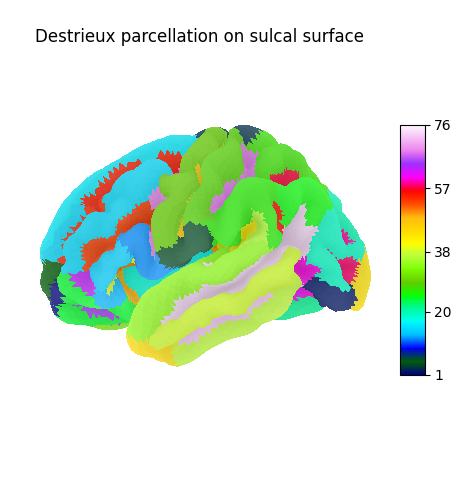`plot_surf_roi`
Plotting surface atlases on a brain surface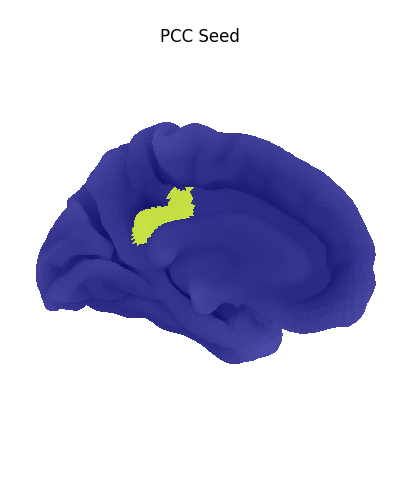`plot_surf_stat_map`
Plotting statistical maps onto a brain surface
Example: Seed-based connectivity on the surface

## 4.7. Interactive plots¶

Nilearn also has functions for making interactive plots that can be seen in a web browser.

New in version 0.5: Interactive plotting is new in nilearn 0.5

For 3D surface plots of statistical maps or surface atlases, use `view_img_on_surf` and `view_surf`. Both produce a 3D plot on the cortical surface. The difference is that `view_surf` takes as input a surface map and a cortical mesh, whereas `view_img_on_surf` takes as input a volume statistical map, and projects it on the cortical surface before making the plot.

For 3D plots of a connectome, use `view_connectome`. To see only markers, use `view_markers`.

### 4.7.1. 3D Plots of statistical maps or atlases on the cortical surface¶

`view_img_on_surf`: Surface plot using a 3D statistical map:

```>>> from nilearn import plotting, datasets
>>> view = plotting.view_img_on_surf(img, threshold='90%', surf_mesh='fsaverage')
```

If you are running a notebook, displaying `view` will embed an interactive plot (this is the case for all interactive plots produced by nilearn’s “view” functions):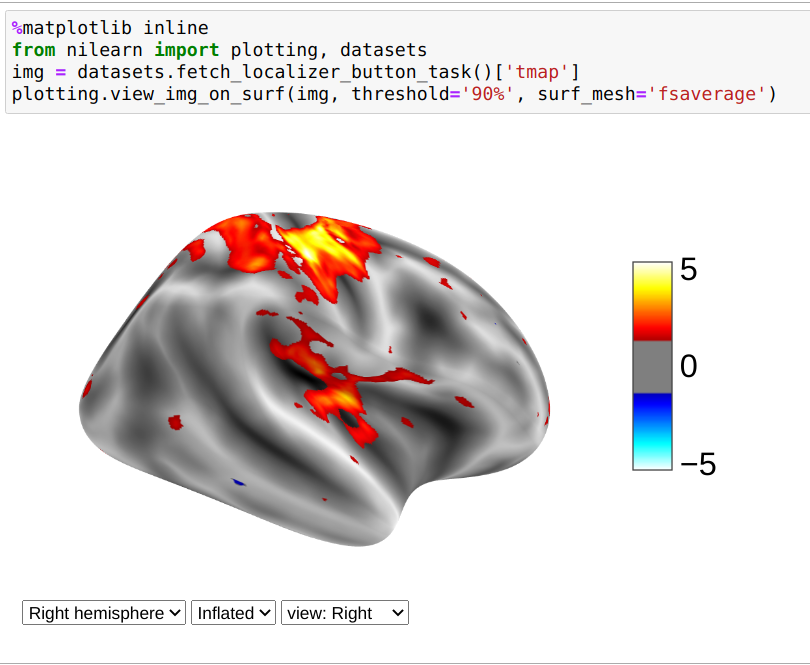If you are not using a notebook, you can open the plot in a browser like this:

```>>> view.open_in_browser()
```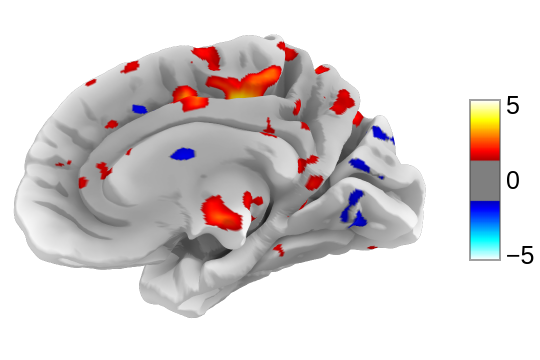Or you can save it to an html file:

```>>> view.save_as_html("surface_plot.html")
```

`view_surf`: Surface plot using a surface map and a cortical mesh:

```>>> from nilearn import plotting, datasets
>>> destrieux = datasets.fetch_atlas_surf_destrieux()
>>> fsaverage = datasets.fetch_surf_fsaverage()
>>> view = plotting.view_surf(fsaverage['infl_left'], destrieux['map_left'],
...                           cmap='gist_ncar', symmetric_cmap=False)
...
>>> view.open_in_browser()
```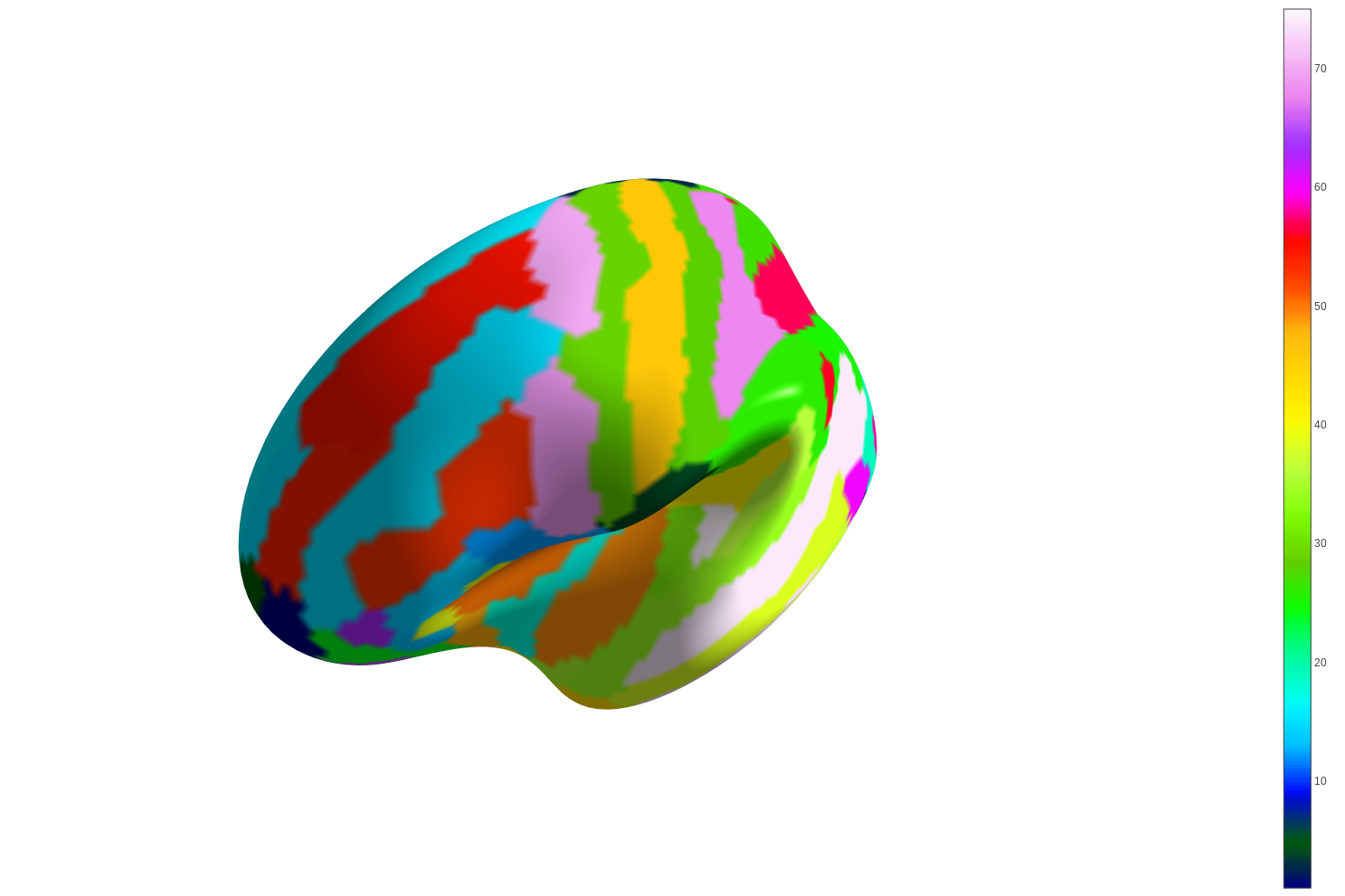### 4.7.2. 3D Plots of connectomes¶

`view_connectome`: 3D plot of a connectome:

```>>> view = plotting.view_connectome(correlation_matrix, coords, edge_threshold='90%')
>>> view.open_in_browser()
```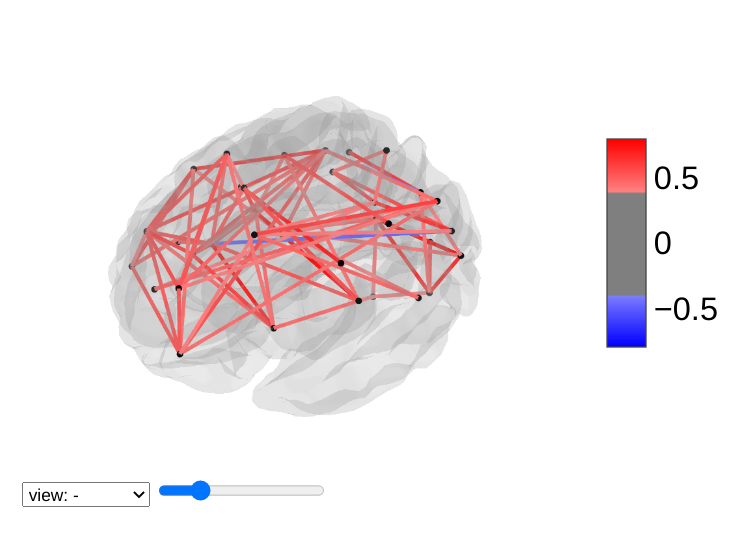### 4.7.3. 3D Plots of markers¶

`view_markers`: showing markers (e.g. seed locations) in 3D:

```>>> from nilearn import plotting
>>> dmn_coords = [(0, -52, 18), (-46, -68, 32), (46, -68, 32), (1, 50, -5)]
>>> view = plotting.view_markers(
>>>       dmn_coords, ['red', 'cyan', 'magenta', 'orange'], marker_size=10)
>>> view.open_in_browser()
```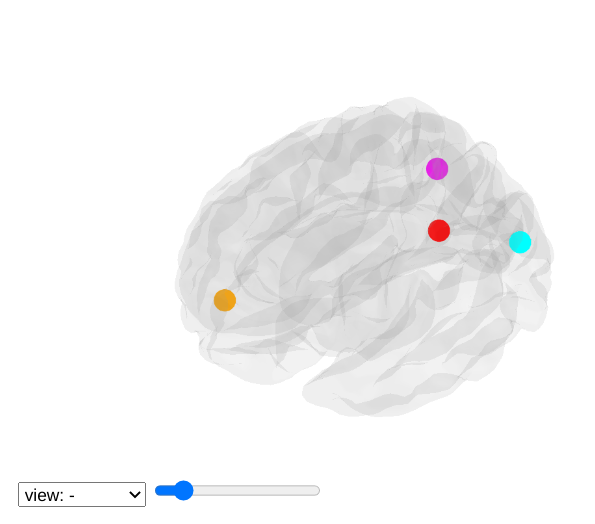### 4.7.4. Interactive visualization of statistical map slices¶

`view_img`: open stat map in a Brainsprite viewer (https://github.com/simexp/brainsprite.js):

```>>> from nilearn import plotting, datasets
>>> html_view = plotting.view_img(img, threshold=2, vmax=4, cut_coords=[-42, -16, 52],
...                                     title="Motor contrast")
```

in a Jupyter notebook, if html_view is not requested, the viewer will be inserted in the notebook: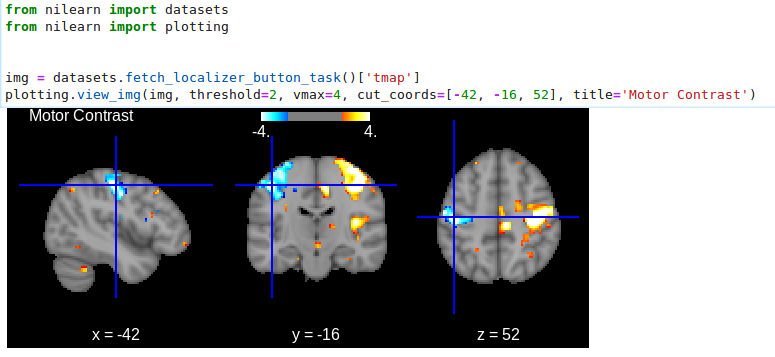```>>> html_view.open_in_browser()
```>>> html_view.save_as_html('viewer.html')